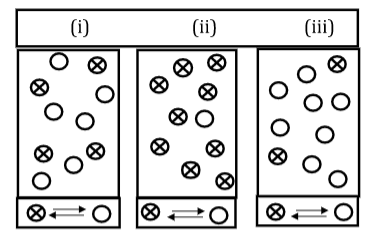# Problem: Each of the following diagrams represents a system at equilibrium at three different temperatures. Rank the three diagrams in order of increasing value for K (assume all chemicals are in gas phase). A) (i) &lt; (ii) &lt; (iii) B) (ii) &lt; (iii) &lt; (i) C) (iii) &lt; (ii) &lt; (i) D) (iii) &lt; (i) &lt; (ii) E) (ii) &lt; (i) &lt; (iii)

###### FREE Expert Solution
91% (101 ratings)
###### Problem Details

Each of the following diagrams represents a system at equilibrium at three different temperatures. Rank the three diagrams in order of increasing value for K (assume all chemicals are in gas phase).

A) (i) < (ii) < (iii)

B) (ii) < (iii) < (i)

C) (iii) < (ii) < (i)

D) (iii) < (i) < (ii)

E) (ii) < (i) < (iii)